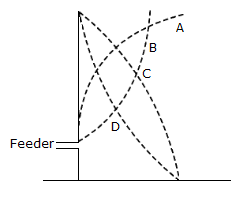# Electronics and Communication Engineering - Electromagnetic Field Theory

1.

 A. loop type B. yagi antenna C. parabolic dishes D. none of the above

Explanation:

No answer description available for this question. Let us discuss.

2.

In figure the current distribution is represented byA. curve A B. curve B C. curve C D. curve D

Explanation:

No answer description available for this question. Let us discuss.

3.

Half power point directions for broadside is

 A. 60° B. 30° C. 45° D. at any angle

Explanation:

No answer description available for this question. Let us discuss.

4.

What will happen to a bird sitting on a conductor?

 A. It will die of shock B. It will not die of shock because its claws are insulated C. It will not die because it is insulated from earth and other conductors D. None of the above

Explanation:

No answer description available for this question. Let us discuss.

5.

Which one is correct option?

 A. Phase velocity is greater than light velocity B. Group velocity is greater than light velocity C. Phase velocity is equal to group velocity D. Phase velocity is less than light velocity